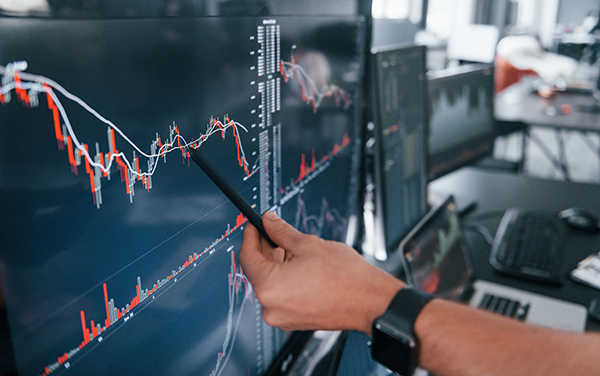# Blog

### Fibonacci Levels in Trading – A Quick IntroThe Fibonacci Levels

The Fibonacci number sequence was discovered by Italian mathematician Leonardo of Pisa or Leonardo Fibonacci. It is one of the most frequently mentioned, and perhaps most frequently understood concepts in technical analysis. Technical traders use Fibonacci levels to determine key buying and selling points, as well as to predict a stock’s upcoming price action.

Today, the Fibonacci levels is taught to most middle-schoolers, but its more complex applications take place in the world of finance. Simply put, the Fibonacci levels are a pattern (starting with 0 and 1) in which each subsequent number is the sum of the previous two.

The numbers are formed by taking the current number and adding it to the previous number in the sequence. 1+1=2; 2+1=3; 3+2=5; 5+3=8; 8+5=13, etc.

Here are the first 20 numbers or so in the sequence: 0, 1, 1, 2, 3, 5, 8, 13, 21, 34, 55, 89, 144, 233, 377, 610, 987, 1597, 2584, 4181, 6765, 10946.

The Fibonacci numbers 13 and 21 are common default values for periods in moving averages and other technical indicators.

The Fibonacci numbers have a common pattern, after you get past the initial couple of numbers:

• Each number in the sequence is 1.618 times the preceding number.
• And, each number is 0.618 times the following number in the sequence.

This ratio is known as known as "the golden ratio" and it appears often in geometry, architecture, art, and in nature.

Fibonacci levels are used in Technical Analysis frequently and based upon the frequency, they have become very important for traders to watch. They are used to measure the magnitude of a trend's "pullback" or "correction." This happens when a trend runs out of momentum and reverses. The trader uses Fibonacci levels to try and identify levels of support and resistance in comparison to the original trend as well as look for levels to enter or exit trades.  Almost all charting platforms feature a Fibonacci drawing tool so draw away!